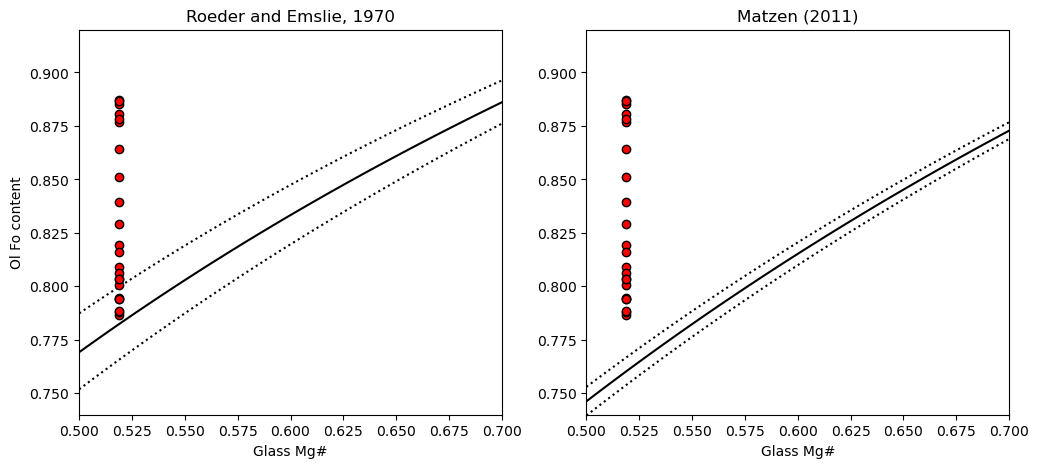This page was generated from docs/Examples/Liquid_Ol_Liq_Themometry/Olivine_MatrixGlass_Mg_Fe_Eq_SingleSamples.ipynb. Interactive online version:.

# 1 Sample - Fe-Mg equilibrium between olivines and matrix glasses

## Install Thermobar if you haven’t already (remove the # and press run!)

:

#!pip install Thermobar

:

# Loading various python things
import numpy as np
import pandas as pd
import matplotlib.pyplot as plt
import Thermobar as pt


### Load in the matrix glasses

:

# We dont have to add _Liq as its all liquids,
# just specify the _Liq in the import
MG_input=pt.import_excel('2018_Olivines_Glasses.xlsx',
sheet_name='LL4_MGs', suffix="_Liq")
MG_all=MG_input['my_input'] ## All columns
MG_Liqs=MG_input['Liqs'] ## Just Liquid columns

:

SiO2_Liq TiO2_Liq Al2O3_Liq FeOt_Liq MnO_Liq MgO_Liq CaO_Liq Na2O_Liq K2O_Liq Cr2O3_Liq P2O5_Liq H2O_Liq Fe3Fet_Liq NiO_Liq CoO_Liq CO2_Liq Sample_ID_Liq
0 50.6243 2.8773 13.0360 11.8288 0.1830 5.8471 10.2471 2.5120 0.5234 0.0 0.2689 0.0 0.0 0.0 0.0 0.0 0
1 50.0975 2.8840 12.8885 11.2897 0.1769 5.8521 9.9658 2.4681 0.5305 0.0 0.2652 0.0 0.0 0.0 0.0 0.0 1
2 50.1346 2.8741 12.8953 11.8696 0.1814 5.8682 10.2012 2.4618 0.5778 0.0 0.2875 0.0 0.0 0.0 0.0 0.0 2
3 50.5233 2.8765 12.9818 11.3536 0.1454 5.8673 10.1806 2.6206 0.5206 0.0 0.2759 0.0 0.0 0.0 0.0 0.0 3
4 51.5840 2.9055 13.3290 11.5014 0.2286 5.8450 10.0408 2.1722 0.5565 0.0 0.2693 0.0 0.0 0.0 0.0 0.0 4

:

Ols_in=pt.import_excel('2018_Olivines_Glasses.xlsx',
sheet_name='LL4_Ols')
Ols_Ol=Ols_in['Ols']
Ols_input=Ols_in['my_input']


## 1. Calculate Mg# for liquids

:

Liq_Mgno_calc=pt.calculate_liq_mgno(liq_comps=MG_Liqs, Fe3Fet_Liq=0.15)
# Calculate mean value to plot
Liq_Mgno_calc_mean=np.mean(Liq_Mgno_calc)


## 2. Calculate Olivine Fo contents

:

Ol_Fo_Calc=pt.calculate_ol_fo(ol_comps=Ols_Ol)


## 3. Calculate fields you want to plot on a Rhodes diagram

:

Rhodes=pt.calculate_ol_rhodes_diagram_lines(Min_Mgno=0.5, Max_Mgno=0.7)

:

Mg#_Liq Eq_Ol_Fo_Roeder (Kd=0.3) Eq_Ol_Fo_Roeder (Kd=0.27) Eq_Ol_Fo_Roeder (Kd=0.33) Eq_Ol_Fo_Matzen (Kd=0.34) Eq_Ol_Fo_Matzen (Kd=0.328) Eq_Ol_Fo_Matzen (Kd=0.352)
0 0.500000 0.769231 0.787402 0.751880 0.746269 0.753012 0.739645
1 0.502020 0.770662 0.788751 0.753384 0.747796 0.754512 0.741198
2 0.504040 0.772087 0.790095 0.754883 0.749317 0.756006 0.742745
3 0.506061 0.773506 0.791432 0.756375 0.750832 0.757493 0.744286
4 0.508081 0.774919 0.792763 0.757861 0.752341 0.758975 0.745822

## 4. Plot the Kd model you want, along with the olivine and glass Mg

:

fig, (ax1, ax2) = plt.subplots(1, 2, figsize=(12,5))

# Plotting for Roeder and Emslie
ax1.set_title('Roeder and Emslie, 1970')
# Plotting equilibrium lines
ax1.plot(Rhodes['Mg#_Liq'], Rhodes['Eq_Ol_Fo_Roeder (Kd=0.27)'], ':k')
ax1.plot(Rhodes['Mg#_Liq'], Rhodes['Eq_Ol_Fo_Roeder (Kd=0.33)'], ':k')
ax1.plot(Rhodes['Mg#_Liq'], Rhodes['Eq_Ol_Fo_Roeder (Kd=0.3)'], '-k')

# Plotting data
ax1.plot(Ol_Fo_Calc*0+Liq_Mgno_calc_mean, Ol_Fo_Calc, 'ok', mfc='red')

ax2.set_title('Matzen (2011)')
# Plotting equilibrium lines
ax2.plot(Rhodes['Mg#_Liq'], Rhodes['Eq_Ol_Fo_Matzen (Kd=0.328)'], ':k')
ax2.plot(Rhodes['Mg#_Liq'], Rhodes['Eq_Ol_Fo_Matzen (Kd=0.352)'], ':k')
ax2.plot(Rhodes['Mg#_Liq'], Rhodes['Eq_Ol_Fo_Matzen (Kd=0.34)'], '-k')
# Plotting data
ax2.plot(Ol_Fo_Calc*0+Liq_Mgno_calc_mean, Ol_Fo_Calc, 'ok', mfc='red')
ax1.set_ylabel('Ol Fo content')
ax1.set_xlabel('Glass Mg#')
ax2.set_xlabel('Glass Mg#')
# You may have to adjust axes limits
ax1.set_ylim([0.74, 0.92])
ax2.set_ylim([0.74, 0.92])
ax1.set_xlim([0.5, 0.7])
ax2.set_xlim([0.5, 0.7])

:

(0.5, 0.7)[ ]:



[ ]:



[ ]: